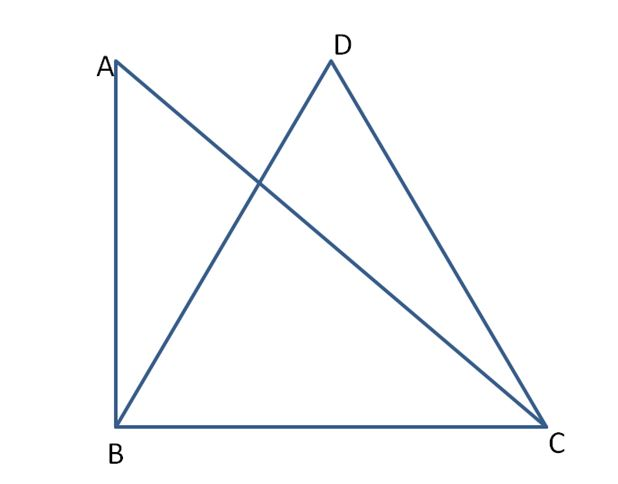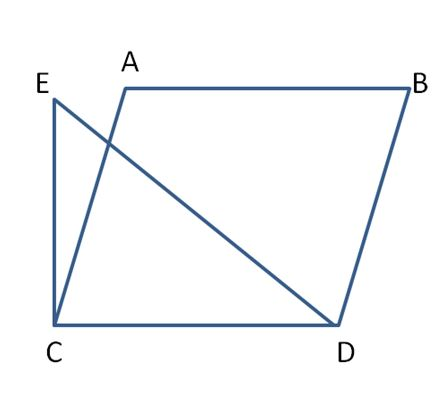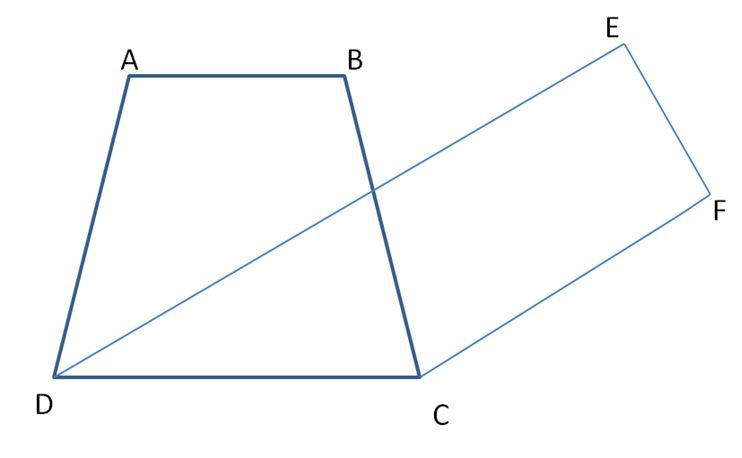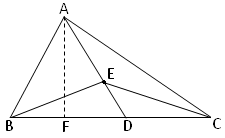# Figures on the Same Base and between Same Parallels | How to Identify Figures on the Same Base and between Same Parallels?

Two geometric shapes are said to be on the same base and between the same parallels, if they have a common side called the base and vertices opposite to the common base on the line parallel to the base. In the below sections, you can learn about the How to Identify figure on the same base and between the same parallels. We know the measure of the plane region enclosed by a closed figure called the area.

The area is measured in cm², m², and other square units. We also know how to calculate the area of different figures using various formulas. Here you will use the formulas to study the relationship between the area of figures when they lie on the same base and between the same parallels.

## Some Geometric Figures on the Same Base and between Same Parallels

Below-mentioned is the geometric figures for which you can change the same base and between the same parallels.

The common base for triangles ABC, BCD is BC. And both triangles lie on the same base.Parallelograms ABCD and triangle CDE lie on the same base CD.Parallelograms ABCD and EFCD are on the same base DC.Trapezium ABCD and parallelogram EFCD have a common side DC. We say that trapezium ABCD and parallelogram EFCD are on the same base DC.### Example Questions on Figures that Lie on the Same Base and Between Same Parallels

Example 1.

ABC is right-angled at B with BC = 10 cm and AC = 15 cm. also ∆ABC and ∆BCD are on the same base BC. Find the area of ∆BCD.Solution:

In right angled ∆ ABC, AC = 15 cm and BC = 10 cm. using Pythagoras theorem, we get

AC² = AB² + BC²

15² = x² + 10²

x² = 15² – 10²

x² = 225 – 100

x² = 125

x = √125

x = 11.18 cm

Now, since ∆ ABC and ∆BCD are on the same base BC.

Therefore, area of ∆ ABC = Area of ∆BCD

1/2 × base × height = Area of ∆BCD

1/2 × 10 × 11.18 = Area of ∆BCD

Area of ∆BCD = 5 x 11.18 = 55.9 cm²

Therefore, area of ∆BCD is 55.9 cm².

Example 2.

AD is the median of ∆ABC. E is any point on AD. Show that area of ∆ABE = area of ∆ACESolution:

The common base for ∆ABE, ∆ACE is AE.

ABC is a triangle with AD as the median.

i.e BD = CD

E is any point on AD.

In ∆ABC,

Given that,

D is the midpoint of BC.

Since median divides the triangle into two triangles of equal area.

So, Area of ∆ABD = Area of ∆ABC —– (i)

In ∆EBC

Given that, D is the midpoint of BC.

∴ ED is median.

Since median divides the triangle into two triangles of equal area.

So, Area of ∆EBD = Area of ∆EDC —— (ii)

Subtracting equation (1) from equation (2)

Area of ∆ABD – Area of ∆EBD = Area of ∆ADC – Area of ∆EDC

Area of ∆ABE = Area of ∆ACE

Hence, proved.

Example 3.

Parallelogram PQRS and PQTU are on the same base PQ and between the same parallels PQ and UR. Area of parallelogram PQRS = 56 cm² and the altitude of the parallelogram PQTU = 7 cm. Find the length of the common side of two parallelograms.Solution:

Given that,

Area of parallelogram PQRS = 56 cm²

The altitude of the parallelogram PQTU = 7 cm

Area of parallelogram PQRS = base x height

56 = PQ x 7

PQ = 56/7

PQ = 8 cm

Therefore, the length of the common side of the two parallelograms is 8 cm.